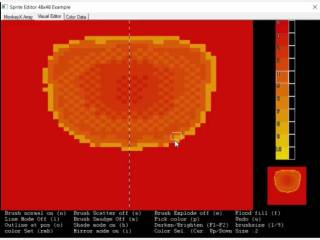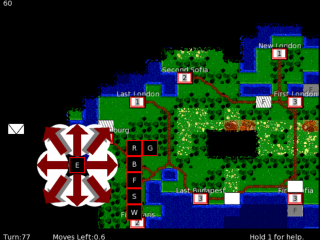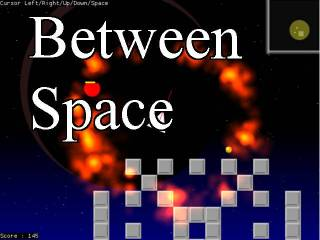-=+=- -=+=- -=+=- -=+=- -=+=- -=+=- -=+=- -=+=- -=+=- -=+=- -=+=- -=+=- -=+=- -=+=- -=+=- -=+=- -=+=- -=+=- -=+=- -=+=- -=+=- -=+=- -=+=- -=+=- -=+=- -=+=- -=+=- -=+=- -=+=- -=+=- (c) WidthPadding Industries 1987 0|460|0 -=+=- -=+=- -=+=- -=+=- -=+=- -=+=- -=+=- -=+=- -=+=- -=+=- -=+=- -=+=- -=+=- -=+=- -=+=- -=+=- -=+=- -=+=- -=+=- -=+=- -=+=- -=+=- -=+=- -=+=- -=+=- -=+=- -=+=- -=+=- -=+=- -=+=-

### Pakz

View Profile, Send Private Message
Recent Posts, Showcases, Articles, Code Snippets, Links, Wed.Workshop, Blog

### Latest Blog Entries

Pakz# Sprite Editor for Monkey2

5th June 2019I started this at noon time. I quite like the idea of having a sprite editor to go with the language I am using. I have yet to <snip>

Pakz# Another All nighter (genetic algorithm)

4th June 2019

Random maps using Genetic Algorithm

Pakz# Platformer with Ropes(emscripten)

3rd June 2019

Nature of code

Pakz# PyxelEdit practise on laptop

1st June 2019

Pixeling with the c64 palette

Pakz# Android pixel art with pixly

26th May 2019I started up pixly on my android phone. I not really used it before. It has advertise<snip>

Pakz# Practising and learning pixel art

15th May 2019

After buying the fantasy console Basic8 I got back into drawing pixel art. I collected a lot of references over the years and this helps a lot. Google also is helpful with the amount of pictures it has.

I have spend 2 days now drawing. I have spend hour<snip>

### Latest Showcases

Pakz# Blitz Basic Sprite Editor

24th August 2018Sprite Editor

Pakz# Fire Power

16th March 2018Fire Power

Pakz# Player vs Zombies or Armed Soldiers

14th October 2017Fight in dungeons against monsters/soldiers

Pakz# A Civilization Clone v0.75

21st June 2017Browser based turn based strategy game

Pakz# Roguelike Explorer

12th April 2017Simple Html5 exploration game with Touch support

Pakz# Between Space

18th December 20162d topdown space shooter with pickups and drones